## OpenIPSL.Electrical.ThreePhase.Branches.Lines

Models for three-phase power lines

### Information

This package contains models for three-phase power lines in different configurations.

Extends from Modelica.Icons.Package (Icon for standard packages).

### Package Content

Name Description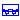Line_1Ph Single-phase line modeled as a PI-equivalent device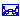Line_2Ph Two-phase line modeled as a PI-equivalent device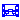Line_3Ph Three-phase line modeled as a PI-equivalent device

##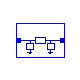OpenIPSL.Electrical.ThreePhase.Branches.Lines.Line_1Ph

Single-phase line modeled as a PI-equivalent device

### Information

This model was design to represent single-phase power line, modeled as a pi-element.

The user should input the series conductance and susceptance, and half shunt susceptance (line charging).

Based on this set of parameters, the pi-equivalent impedance matrices are calculated and the power line equation is assembled.

Extends from Branches.BaseClasses.baseLine (Partial base power line model).

### Parameters

NameDescription
Power flow data
SNominal Power [VA]
fSystem Frequency [Hz]
GserSeries conductance 
BserSeries susceptance 
BshtShunt half susceptance 

NameDescription
Ain
Aout

##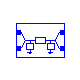OpenIPSL.Electrical.ThreePhase.Branches.Lines.Line_2Ph

Two-phase line modeled as a PI-equivalent device

### Information

This model was design to represent two-phase power line, modeled as a pi-element.

The user should input the series conductance and susceptance, and half shunt susceptance (line charging). All in matrix form, since the model allows the representation of unbalanced lines. Series conductance (Gser) matrix is considered to have the following structure:

[Gseraa, Gserab;

Gserab, Gserbb]

Series susceptance (Bser) matrix is considered to have the following structure:

[Bseraa, Bserab;

Bserab, Bserbb]

The series admittance matrix is Yser = Gser+jBser. Each of the two shunt susceptance (Bsht) matrices is considered to have the following structure:

[Bshtaa, Bshtab;

Bshtab, Bshtbb]

Based on this set of parameters, the pi-equivalent impedance matrices are calculated and the power line equation is assembled.

Extends from Branches.BaseClasses.baseLine (Partial base power line model).

### Parameters

NameDescription
Power flow data
SNominal Power [VA]
fSystem Frequency [Hz]
GseraaElement (1,1) in series conductance matrix 
BseraaElement (1,1) in series susceptance matrix 
GserabElement (1,2) in series conductance matrix 
BserabElement (1,2) in series susceptance matrix 
GserbbElement (2,2) in series conductance matrix 
BserbbElement (2,2) in series susceptance matrix 
BshtaaElement (1,1) in shunt half susceptance matrix 
BshtabElement (1,2) in shunt half susceptance matrix 
BshtbbElement (2,2) in shunt half susceptance matrix 

NameDescription
Ain
Bin
Aout
Bout

##OpenIPSL.Electrical.ThreePhase.Branches.Lines.Line_3Ph

Three-phase line modeled as a PI-equivalent device

### Information

This model was design to represent three-phase power line, modeled as a pi-element.

The user should input the series conductance and susceptance, and half shunt susceptance (line charging). All in matrix form, since the model allows the representation of unbalanced lines. Series conductance (Gser) matrix is considered to have the following structure:

[Gseraa, Gserab, Gserac;

Gserab, Gserbb, Gserbc;

Gserac, Gserbc, Gsercc]

Series susceptance (Bser) matrix is considered to have the following structure:

[Bseraa, Bserab, Bserac;

Bserab, Bserbb, Bserbc;

Bserac, Bserbc, Bsercc]

The series admittance matrix is Yser = Gser+jBser. Each of the two shunt susceptance (Bsht) matrices is considered to have the following structure:

[Bshtaa, Bshtab, Bshtac;

Bshtab, Bshtbb, Bshtbc;

Bshtac, Bshtbc, Bshtcc]

Based on this set of parameters, the pi-equivalent impedance matrices are calculated and the power line equation is assembled.

Extends from ThreePhase.Branches.BaseClasses.baseLine (Partial base power line model).

### Parameters

NameDescription
Power flow data
SNominal Power [VA]
fSystem Frequency [Hz]
GseraaElement (1,1) in series conductance matrix 
BseraaElement (1,1) in series susceptance matrix 
GserabElement (1,2) in series conductance matrix 
BserabElement (1,2) in series susceptance matrix 
GseracElement (1,3) in series conductance matrix 
BseracElement (1,3) in series susceptance matrix 
GserbbElement (2,2) in series conductance matrix 
BserbbElement (2,2) in series susceptance matrix 
GserbcElement (2,3) in series conductance matrix 
BserbcElement (2,3) in series susceptance matrix 
GserccElement (3,3) in series conductance matrix 
BserccElement (3,3) in series susceptance matrix 
BshtaaElement (1,1) in shunt half susceptance matrix 
BshtabElement (1,2) in shunt half susceptance matrix 
BshtacElement (1,3) in shunt half susceptance matrix 
BshtbbElement (2,2) in shunt half susceptance matrix 
BshtbcElement (2,3) in shunt half susceptance matrix 
BshtccElement (3,3) in shunt half susceptance matrix 

### Connectors

NameDescription
Ain
Bin
Cin
Aout
Bout
Cout
Automatically generated Thu Oct 6 17:41:44 2022.